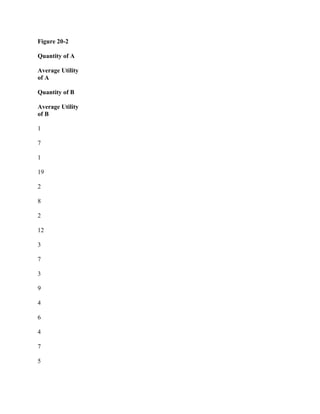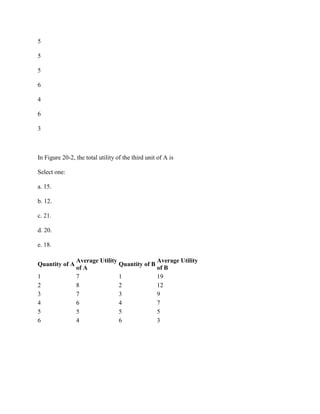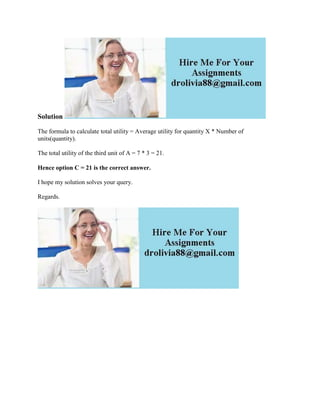Seu SlideShare está sendo baixado. ×

# Figure 20-2 Quantity of A Average Utility of A Quantity of B Average U.docx

Anúncio
Anúncio
Anúncio
Anúncio
Anúncio
Anúncio
Anúncio
Anúncio
Anúncio
Anúncio
AnúncioCarregando em…3
×

1 de 3 Anúncio

# Figure 20-2 Quantity of A Average Utility of A Quantity of B Average U.docx

Figure 20-2
Quantity of A
Average Utility
of A
Quantity of B
Average Utility
of B
1
7
1
19
2
8
2
12
3
7
3
9
4
6
4
7
5
5
5
5
6
4
6
3
In Figure 20-2, the total utility of the third unit of A is
Select one:
a. 15.
b. 12.
c. 21.
d. 20.
e. 18.
Solution
The formula to calculate total utility = Average utility for quantity X * Number of units(quantity).
The total utility of the third unit of A = 7 * 3 = 21.
Hence option C = 21 is the correct answer.
I hope my solution solves your query.
Regards.
.

Figure 20-2
Quantity of A
Average Utility
of A
Quantity of B
Average Utility
of B
1
7
1
19
2
8
2
12
3
7
3
9
4
6
4
7
5
5
5
5
6
4
6
3
In Figure 20-2, the total utility of the third unit of A is
Select one:
a. 15.
b. 12.
c. 21.
d. 20.
e. 18.
Solution
The formula to calculate total utility = Average utility for quantity X * Number of units(quantity).
The total utility of the third unit of A = 7 * 3 = 21.
Hence option C = 21 is the correct answer.
I hope my solution solves your query.
Regards.
.

Anúncio
Anúncio

### Figure 20-2 Quantity of A Average Utility of A Quantity of B Average U.docx

1. 1. Figure 20-2 Quantity of A Average Utility of A Quantity of B Average Utility of B 1 7 1 19 2 8 2 12 3 7 3 9 4 6 4 7 5
2. 2. 5 5 5 6 4 6 3 In Figure 20-2, the total utility of the third unit of A is Select one: a. 15. b. 12. c. 21. d. 20. e. 18. Quantity of A Average Utility of A Quantity of B Average Utility of B 1 7 1 19 2 8 2 12 3 7 3 9 4 6 4 7 5 5 5 5 6 4 6 3
3. 3. Solution The formula to calculate total utility = Average utility for quantity X * Number of units(quantity). The total utility of the third unit of A = 7 * 3 = 21. Hence option C = 21 is the correct answer. I hope my solution solves your query. Regards.